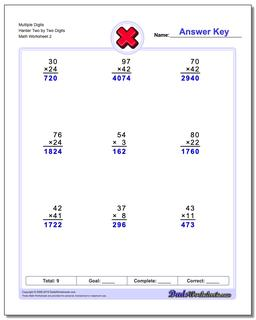PLEASE GO BACK AND USE THE BIG BLUE 'PRINT' BUTTON ON THE PAGE TO PRINT THE WORKSHEET CORRECTLY!Sorry for the trouble! The browser won't print the embedded worksheet PDF directly using the normal 'Print' command in the file menu, so you need to click the big 'Print' button to send just the worksheet and not the surrounding page to the printer.

# Math Worksheets: Multiplication: Multiplication: Multiple Digits Harder Two by Two Digits (Second Worksheet)## Multiple Digits Harder Two by Two Digits (Second Worksheet)

PropertyValue
DescriptionMultiple Digits Harder Two by Two Digits: These multiplication worksheets introduce two digit and three digit multiplication problems. Try out this multiplication calculator to see interactively how to do long multiplication. (Second Worksheet)
Resource TypeWorksheet